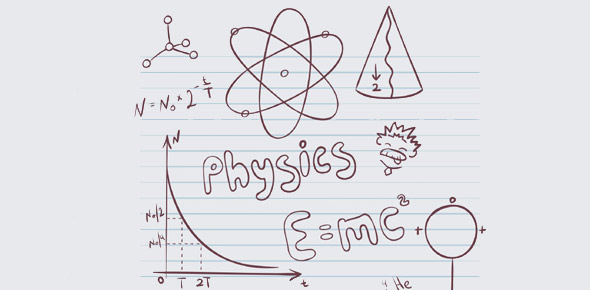# Physics Test #1 Chapter 2 Part 1

29 Questions | Total Attempts: 55Settings• 1.
A wave is a traveling variation in quantities called?
• 2.
Sound is a traveling variation in quantities called?
• 3.
Ultrasound is a sound with a frequency greater than ________Hz
• 4.
Acoustic Variables include __________, __________, and __________ _________*3 Variables*
• A.

Wave

• B.

Pressure

• C.

Density

• D.

Particle Vibration

• 5.
Which of the following frequencies are in the Ultrasound Range?
• A.

15 Hz

• B.

15,000 Hz

• C.

15 MHz

• D.

30,000Hz

• E.

0.04 MHz

• 6.
Which of the following are Acoustic Variables? ***More than one correct answer***
• A.

Pressure

• B.

Frequecy

• C.

Propagation Speed

• D.

Particle Motion

• E.

Period

• 7.
Frequency is a measure of how many ______ an acoustic variable goes through in a second.
• 8.
The unit of frequency is ______, which is abbreviated________
• 9.
Period is the ______ that it takes for one cycle to occur.
• 10.
Period decreases as ____________ increases.
• 11.
Wavelength is the length of ______ over which one cycle occurs
• 12.
Propagation Speed is the speed with which a ________ moves through a medium in 1 second.
• 13.
Wavelength is equal to _________ ________ divided by _________
• 14.
The _________ and_________ of a medium determine Propagation Speed
• 15.
Propagation Speed Increases if ____________ is Increased.
• 16.
The average Propagation Speed in soft tissues is ?
• A.

1,540 m/s

• B.

1,540 mm/us

• C.

1.54 m/s

• D.

1.54 mm/us

• 17.
Propagation Speed is determined by
• A.

Frequency

• B.

Amplitude

• C.

Wavelength

• D.

Period

• E.

Medium

• 18.
The wavelength of 7-MHz ultrasound in soft tissue is __________mm
• 19.
Wavelength in Soft Tissue _________ as Frequency Increases
• 20.
It takes ________us for ultrasound to travel 1.54cm  in Soft Tissue
• 21.
Propagation Speed in Bone is ______ than in Soft Tissue.
• 22.
Sound travels fastest in?
• A.

Air

• B.

Helium

• C.

Water

• D.

Steel

• E.

A Vacuum

• 23.
Solids have higher propagation speeds than liquids because they have greater
• A.

Density

• B.

Stiffness

• 24.
Sounds travels slowest in?
• A.

Gases

• B.

Steel

• 25.
Sound is a _____________wave *More than 1 correct answer*
• A.

Mechanical

• B.

Longitudinal

• C.

Compressional

Related TopicsBack to top